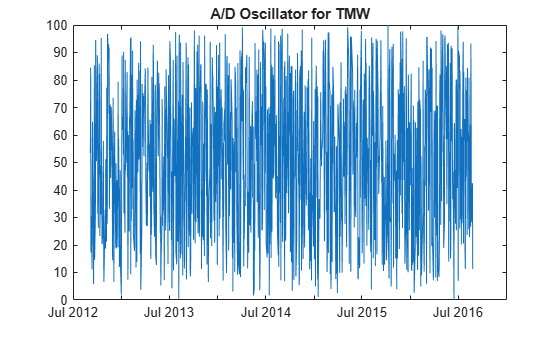Main Content

# adosc

Accumulation/Distribution oscillator

Using a `fints` object for the `Data` argument of `adosc` is not recommended. Use a matrix, `timetable`, or `table` instead for financial time series. For more information, see Convert Financial Time Series Objects fints to Timetables.

## Syntax

``ado = adosc(Data)``

## Description

example

````ado = adosc(Data)` calculates the Accumulation/Distribution (A/D) oscillator.```

## Examples

collapse all

Load the file `SimulatedStock.mat`, which provides a timetable (`TMW`) for financial data for TMW stock.

```load SimulatedStock.mat ADOsc = adosc(TMW); plot(ADOsc.dates, ADOsc.ADOscillator) title('A/D Oscillator for TMW')```## Input Arguments

collapse all

Data with open, high, low, close information, specified as a matrix, table, or timetable. For matrix input, `Data` is an `M`-by-`4` matrix of open, high, low, and closing prices. Timetables and tables with `M` rows must contain variables named `'Open'`, `'High'`, `'Low'`, and `'Close'` (case insensitive).

Data Types: `double` | `table` | `timetable`

## Output Arguments

collapse all

Accumulation/Distribution oscillator, returned with the same number of rows (`M`) and type (matrix, table, or timetable) as the input `Data`.

 Kaufman, P. J. The New Commodity Trading Systems and Methods. John Wiley and Sons, New York, 1987.

Download ebook